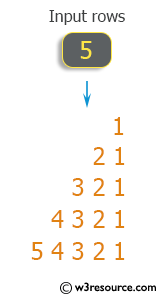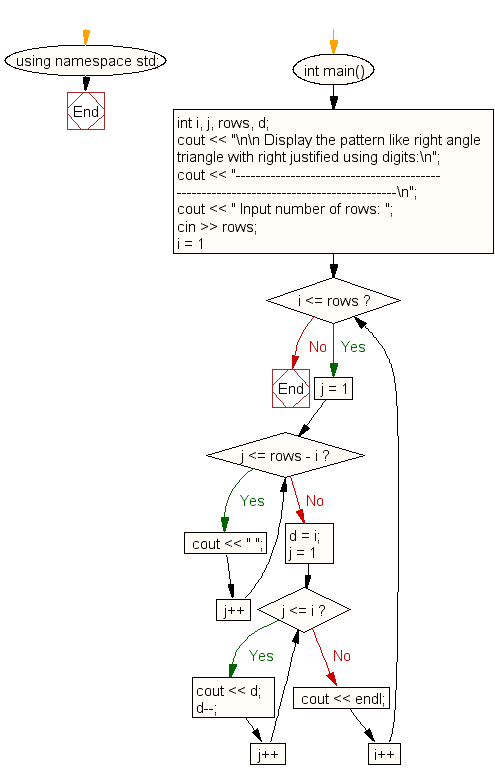﻿ C++ : Pattern like right angle triangle with right justified

# C++ Exercises: Display the pattern like right angle triangle with right justified using digits

## C++ For Loop: Exercise-53 with Solution

Write a C++ program to display the pattern like right angle triangle with right justified digits.

Pictorial Presentation:Sample Solution:

C++ Code :

``````#include <iostream>
using namespace std;

int main()
{
int i, j, rows, d;
cout << "\n\n Display the pattern like right angle triangle with right justified using digits:\n";
cout << "-------------------------------------------------------------------------------------\n";
cout << " Input number of rows: ";
cin >> rows;
for (i = 1; i <= rows; i++)
{
for (j = 1; j <= rows - i; j++)
{
cout << " ";
}
d = i;
for (j = 1; j <= i; j++)
{
cout << d;
d--;
}
cout << endl;
}
}
``````

Sample Output:

``` Display the pattern like right angle triangle with right justified using digits:
-------------------------------------------------------------------------------------
Input number of rows: 5
1
21
321
4321
54321
```

Flowchart:C++ Code Editor: